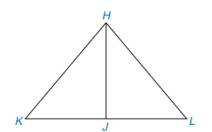Chapter 3.2, Problem 13E### Elementary Geometry for College St...

6th Edition
Daniel C. Alexander + 1 other
ISBN: 9781285195698

#### Solutions

Chapter
Section### Elementary Geometry for College St...

6th Edition
Daniel C. Alexander + 1 other
ISBN: 9781285195698
Textbook Problem
1 views

# In Exercise 13and 14, complete each proof. Given: H J ¯ ⊥ K L ¯ and H K ¯ ≅ H L ¯ (See figure below)Prove: K J ¯ ≅ J L ¯ PROOF Statements Reasons 1. H J ¯ ⊥ K L ¯ and H K ¯ ≅ H L ¯ 1. ? 2. ∠ s H J K and H J L are rt ∠ s 2. ? 3. H J ¯ ≅ H J ¯ 3. ? 4. ? 4. HL 5. ? 5. CPCTC

To determine

To prove:

The given statement.

Explanation

Given:

The following figure shows the given diagram.

Given that,

HJ¯KL¯ and HK¯HL¯.

Approach:

By definition of hypotenuse leg congruency (HL), if two triangles have corresponding sides that are then triangle will be congruent.

The completed proof is shown in the following table.

 PROOF Statements Reasons 1. HJ¯⊥KL¯ and HK¯≅HL¯ 1. Given 2.∠s HJK and HJL are right ∠s 2.HJ¯⊥KL¯ 3.HJ¯≅HJ¯ 3. Identity 4. ΔHJK≅ΔHJL 4

### Still sussing out bartleby?

Check out a sample textbook solution.

See a sample solution

#### The Solution to Your Study Problems

Bartleby provides explanations to thousands of textbook problems written by our experts, many with advanced degrees!

Get Started